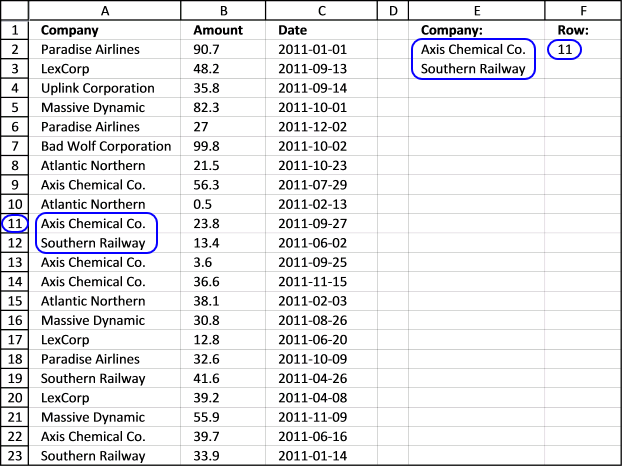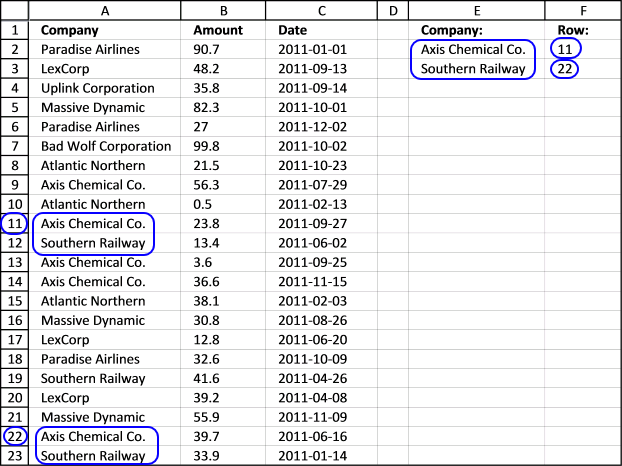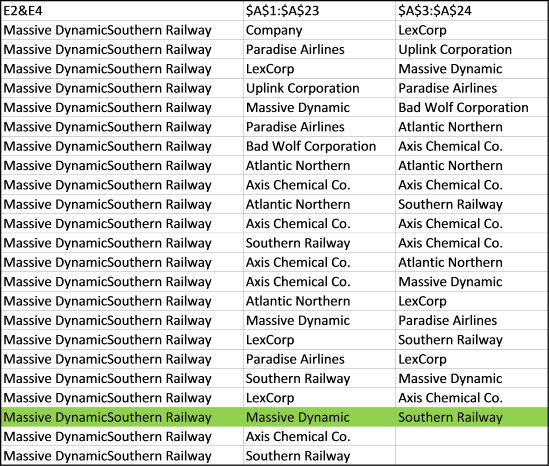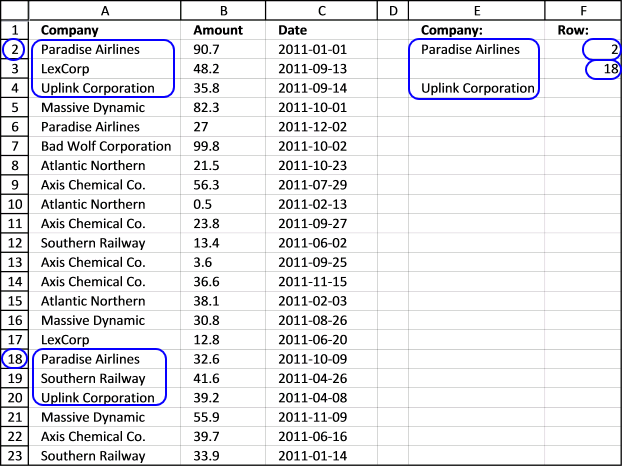Author: Oscar Cronquist Article last updated on July 29, 2017

This array formula finds the sequence "Axis Chemical Co." (cell E2) and "Southern Railway" (cell E3) in two consecutive cells in column A and returns the row number in cell F2.Array formula in cell F11:

=MATCH(E2&E3,\$A\$1:\$A\$23&\$A\$2:\$A\$24,0)

The second argument in the MATCH function is \$A\$1:\$A\$23&\$A\$2:\$A\$24. The ampersand concatenates the two cell ranges into one. By concatenating a value with the value below you can easily search for a sequence of values.
How to enter an array formula

1. Select cell F2
2. Click in the formula bar
3. Paste formula to formula bar
4. Press and hold CTRL + SHIFT
5. Press Enter once
6. Release all keys

### Find multiple sequencesArray formula in cell F2:

=SMALL(IF(\$E\$2&\$E\$3=\$A\$1:\$A\$23&\$A\$2:\$A\$24, MATCH(ROW(\$A\$2:\$A\$23), ROW(\$A\$2:\$A\$23))), ROW(A1))

Copy cell F2 and paste cells below as far as needed.

### Find a sequence with any value betweenArray formula in cell F2:

=MATCH(E2&E4,\$A\$1:\$A\$23&\$A\$3:\$A\$24,0)

If you examine this combined cell ref \$A\$1:\$A\$23&\$A\$3:\$A\$25 you can see that the first one start at A1 and the second one at A3. This is because the second value in the sequence is a value that can be anything. Let us see the values behind these cell refs.### Find multiple sequences with any value betweenArray formula in cell F2:

=SMALL(IF(\$E\$2&\$E\$4=\$A\$1:\$A\$23&\$A\$3:\$A\$25, MATCH(ROW(\$A\$2:\$A\$23), ROW(\$A\$2:\$A\$23))), ROW(A1))

### Finding a sequence - potential problems

=MATCH(E2&E3,B1:B23&B2:B24,0)

As you can see, the formula returns row 2. Why is that? The MATCH function looks for the value in cell E2 and E3 combined like this, 53 and 89 becomes 5389. The values in cell B2 and B3 are 538 and 9, becomes 5389 and that is a match.

So if we add a delimiting character we can rule out these problems, see the next formula.

Array formula in cell F6:

=MATCH(E2&"-"&E3,B1:B23&"-"&B2:B24,0)

But to be really sure that this works you must check that the character "-" does not exist at all in column B or you could get same the error again. The next formula uses the COUNTIFS function and I think this is the best method, no need for delimiting characters.

Array formula in cell F10:

=MATCH(1,COUNTIFS(E10,B1:B23,E11,B2:B24),0)

The downside with this formula is that you can´t find multiple sequences in a column.